# RD Sharma Solutions for Class 6 Maths Chapter 9: Ratio, Proportion and Unitary Method Exercise 9.3

RD Sharma Solutions Class 6 Exercise 9.3 helps students understand concepts like proportion and problems based on them. An equality of two ratios is called a proportion. The two types of proportions are continued proportion and mean proportion. The solutions prepared by experts in Mathematics help students to score well in the annual exam. The students can download RD Sharma Solutions for Class 6 Maths Chapter 9 Ratio, Proportion and Unitary Method Exercise 9.3 PDF which are provided here.

## RD Sharma Solutions for Class 6 Maths Chapter 9: Ratio, Proportion and Unitary Method Exercise 9.3 Download PDF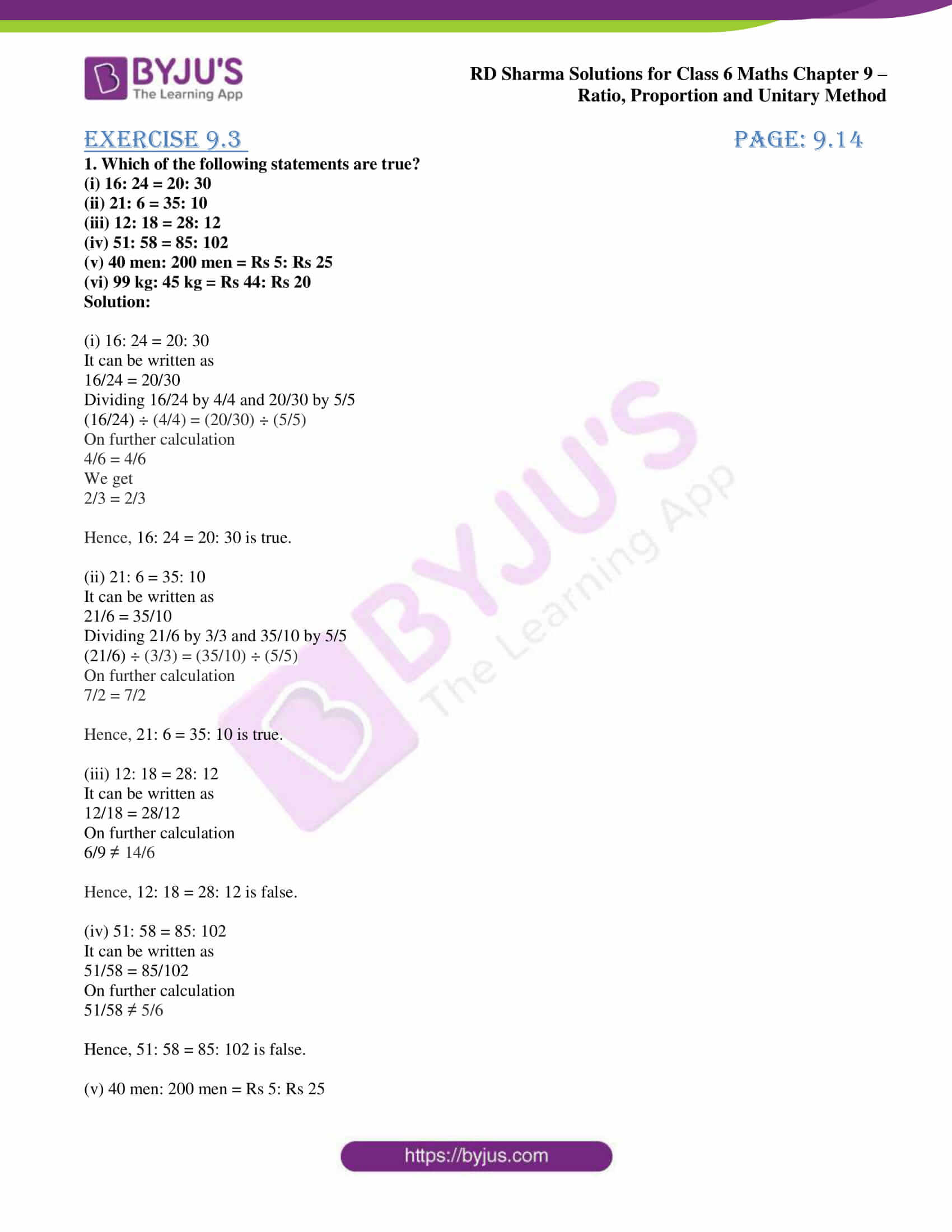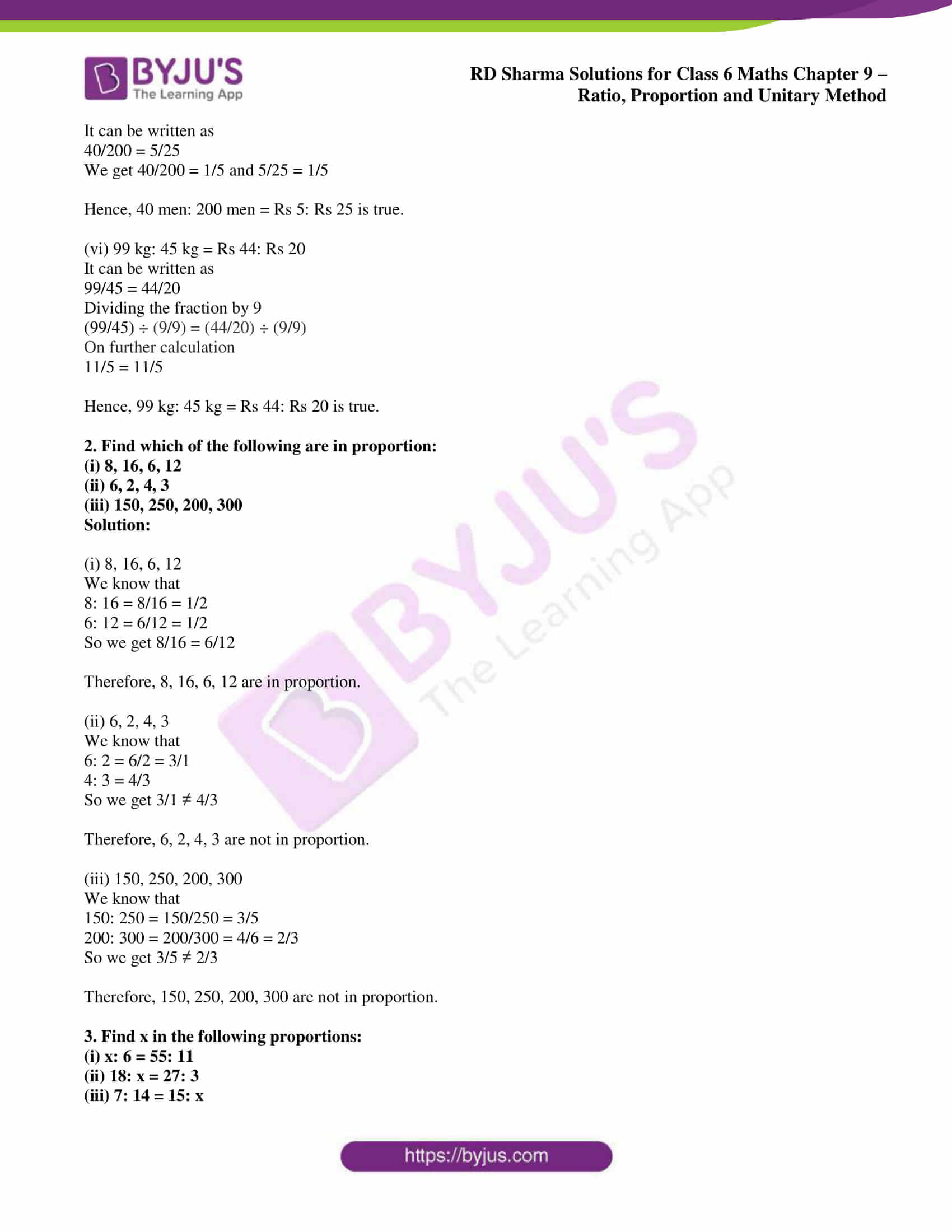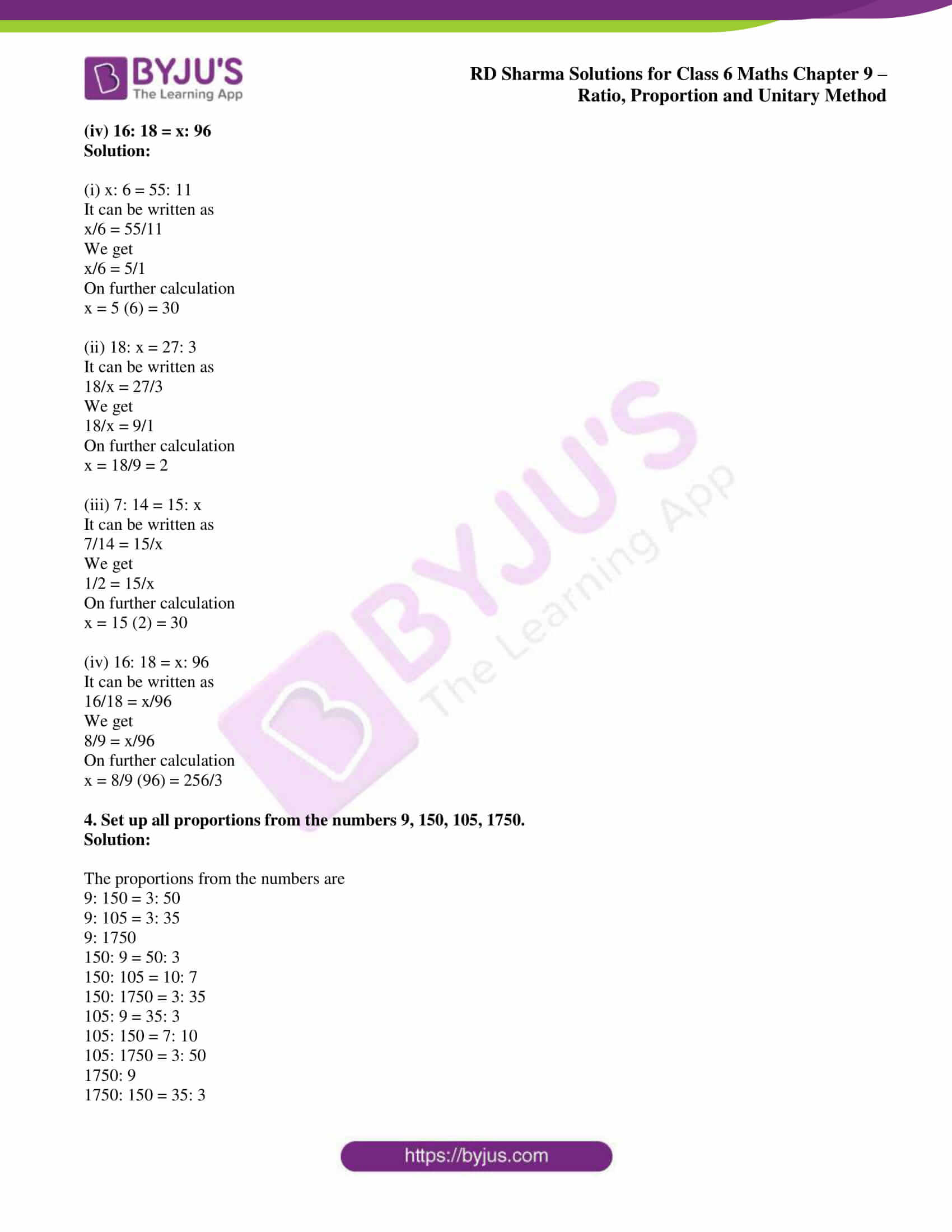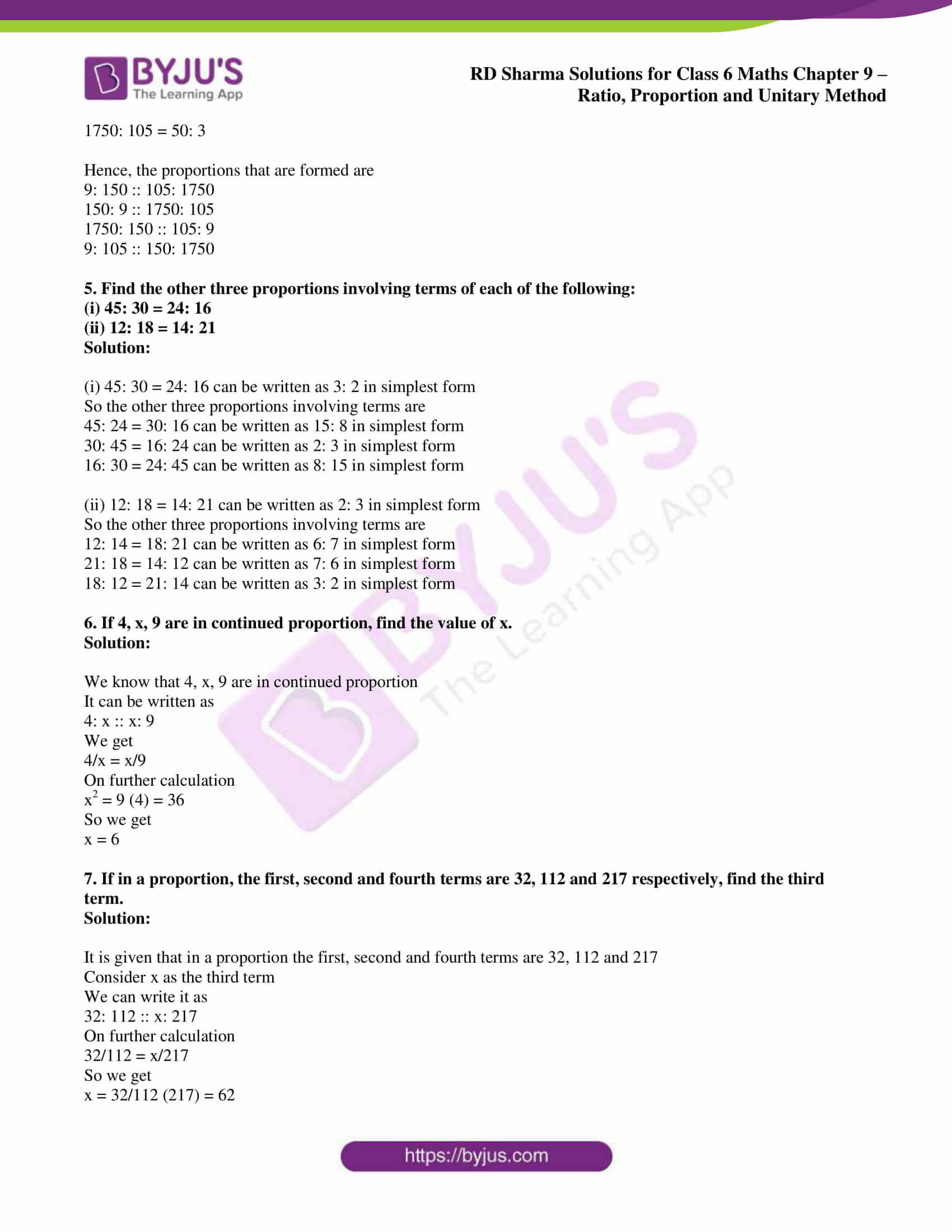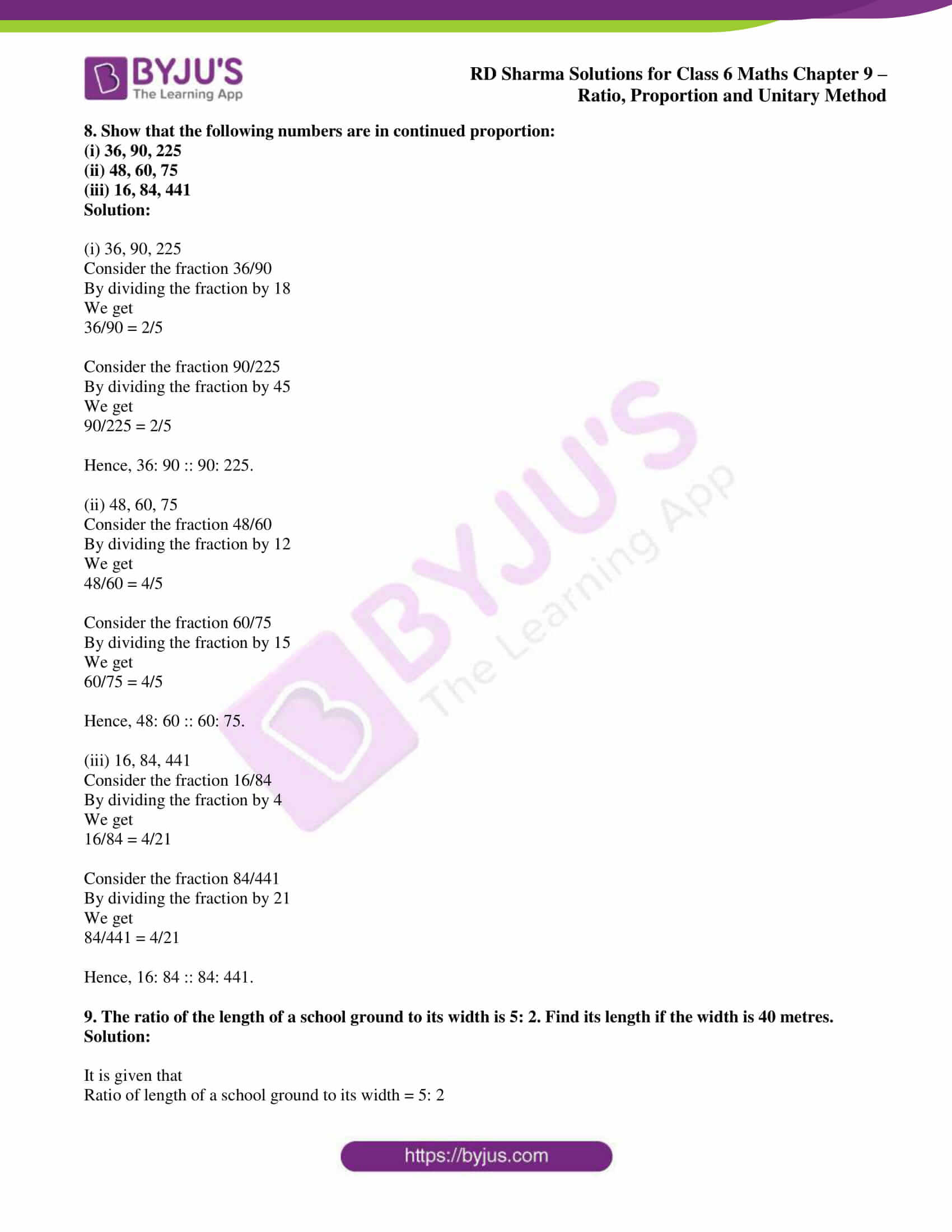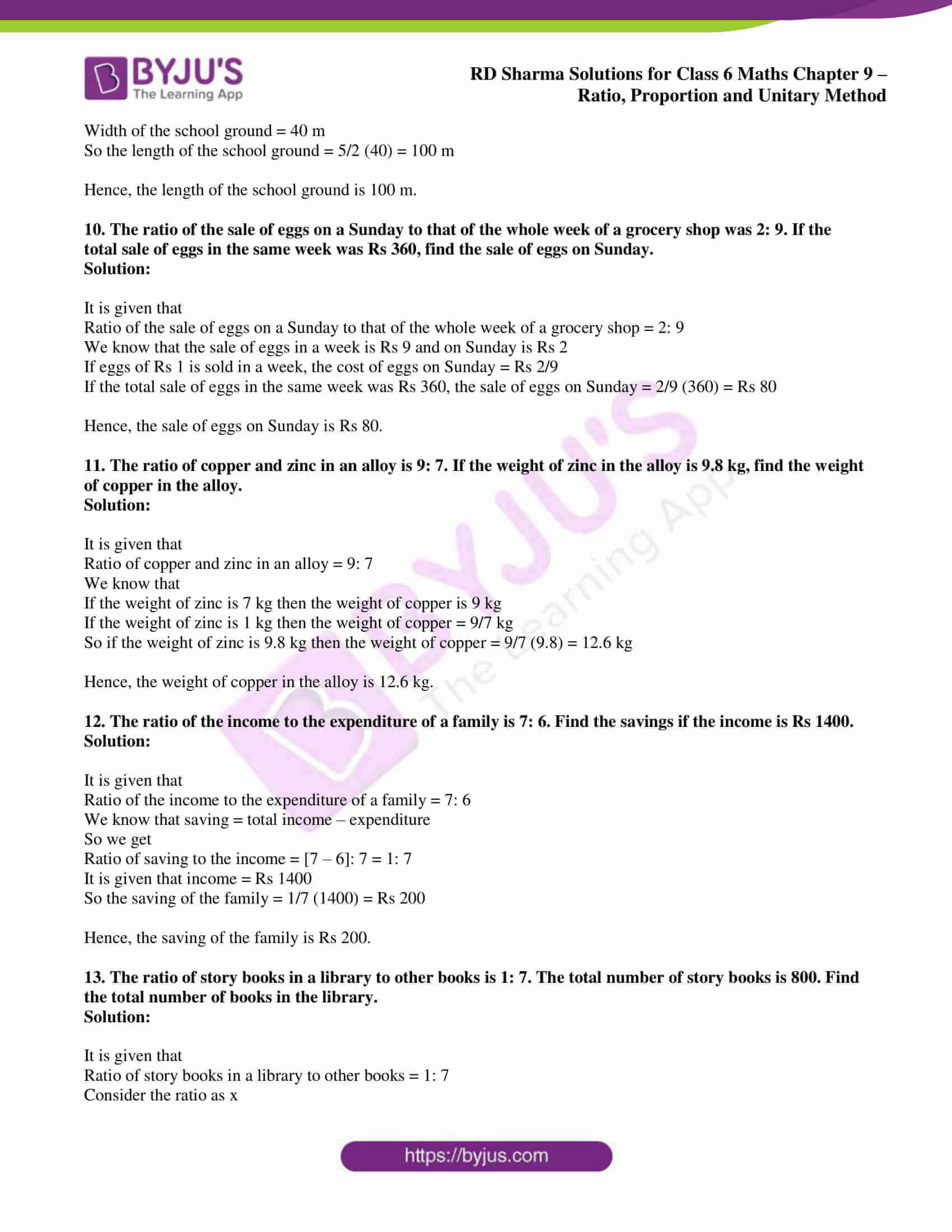### Access answers to Maths RD Sharma Solutions for Class 6 Chapter 9: Ratio, Proportion and Unitary Method Exercise 9.3

1. Which of the following statements are true?

(i) 16: 24 = 20: 30

(ii) 21: 6 = 35: 10

(iii) 12: 18 = 28: 12

(iv) 51: 58 = 85: 102

(v) 40 men: 200 men = Rs 5: Rs 25

(vi) 99 kg: 45 kg = Rs 44: Rs 20

Solution:

(i) 16: 24 = 20: 30

It can be written as

16/24 = 20/30

Dividing 16/24 by 4/4 and 20/30 by 5/5

(16/24) ÷ (4/4) = (20/30) ÷ (5/5)

On further calculation

4/6 = 4/6

We get

2/3 = 2/3

Hence, 16: 24 = 20: 30 is true.

(ii) 21: 6 = 35: 10

It can be written as

21/6 = 35/10

Dividing 21/6 by 3/3 and 35/10 by 5/5

(21/6) ÷ (3/3) = (35/10) ÷ (5/5)

On further calculation

7/2 = 7/2

Hence, 21: 6 = 35: 10 is true.

(iii) 12: 18 = 28: 12

It can be written as

12/18 = 28/12

On further calculation

6/9 ≠ 14/6

Hence, 12: 18 = 28: 12 is false.

(iv) 51: 58 = 85: 102

It can be written as

51/58 = 85/102

On further calculation

51/58 ≠ 5/6

Hence, 51: 58 = 85: 102 is false.

(v) 40 men: 200 men = Rs 5: Rs 25

It can be written as

40/200 = 5/25

We get 40/200 = 1/5 and 5/25 = 1/5

Hence, 40 men: 200 men = Rs 5: Rs 25 is true.

(vi) 99 kg: 45 kg = Rs 44: Rs 20

It can be written as

99/45 = 44/20

Dividing the fraction by 9

(99/45) ÷ (9/9) = (44/20) ÷ (9/9)

On further calculation

11/5 = 11/5

Hence, 99 kg: 45 kg = Rs 44: Rs 20 is true.

2. Find which of the following are in proportion:

(i) 8, 16, 6, 12

(ii) 6, 2, 4, 3

(iii) 150, 250, 200, 300

Solution:

(i) 8, 16, 6, 12

We know that

8: 16 = 8/16 = 1/2

6: 12 = 6/12 = 1/2

So we get 8/16 = 6/12

Therefore, 8, 16, 6, 12 are in proportion.

(ii) 6, 2, 4, 3

We know that

6: 2 = 6/2 = 3/1

4: 3 = 4/3

So we get 3/1 ≠ 4/3

Therefore, 6, 2, 4, 3 are not in proportion.

(iii) 150, 250, 200, 300

We know that

150: 250 = 150/250 = 3/5

200: 300 = 200/300 = 4/6 = 2/3

So we get 3/5 ≠ 2/3

Therefore, 150, 250, 200, 300 are not in proportion.

3. Find x in the following proportions:

(i) x: 6 = 55: 11

(ii) 18: x = 27: 3

(iii) 7: 14 = 15: x

(iv) 16: 18 = x: 96

Solution:

(i) x: 6 = 55: 11

It can be written as

x/6 = 55/11

We get

x/6 = 5/1

On further calculation

x = 5 (6) = 30

(ii) 18: x = 27: 3

It can be written as

18/x = 27/3

We get

18/x = 9/1

On further calculation

x = 18/9 = 2

(iii) 7: 14 = 15: x

It can be written as

7/14 = 15/x

We get

1/2 = 15/x

On further calculation

x = 15 (2) = 30

(iv) 16: 18 = x: 96

It can be written as

16/18 = x/96

We get

8/9 = x/96

On further calculation

x = 8/9 (96) = 256/3

4. Set up all proportions from the numbers 9, 150, 105, 1750.

Solution:

The proportions from the numbers are

9: 150 = 3: 50

9: 105 = 3: 35

9: 1750

150: 9 = 50: 3

150: 105 = 10: 7

150: 1750 = 3: 35

105: 9 = 35: 3

105: 150 = 7: 10

105: 1750 = 3: 50

1750: 9

1750: 150 = 35: 3

1750: 105 = 50: 3

Hence, the proportions that are formed are

9: 150 :: 105: 1750

150: 9 :: 1750: 105

1750: 150 :: 105: 9

9: 105 :: 150: 1750

5. Find the other three proportions involving terms of each of the following:

(i) 45: 30 = 24: 16

(ii) 12: 18 = 14: 21

Solution:

(i) 45: 30 = 24: 16 can be written as 3: 2 in simplest form

So the other three proportions involving terms are

45: 24 = 30: 16 can be written as 15: 8 in simplest form

30: 45 = 16: 24 can be written as 2: 3 in simplest form

16: 30 = 24: 45 can be written as 8: 15 in simplest form

(ii) 12: 18 = 14: 21 can be written as 2: 3 in simplest form

So the other three proportions involving terms are

12: 14 = 18: 21 can be written as 6: 7 in simplest form

21: 18 = 14: 12 can be written as 7: 6 in simplest form

18: 12 = 21: 14 can be written as 3: 2 in simplest form

6. If 4, x, 9 are in continued proportion, find the value of x.

Solution:

We know that 4, x, 9 are in continued proportion

It can be written as

4: x :: x: 9

We get

4/x = x/9

On further calculation

x2 = 9 (4) = 36

So we get

x = 6

7. If in a proportion, the first, second and fourth terms are 32, 112 and 217 respectively, find the third term.

Solution:

It is given that in a proportion the first, second and fourth terms are 32, 112 and 217

Consider x as the third term

We can write it as

32: 112 :: x: 217

On further calculation

32/112 = x/217

So we get

x = 32/112 (217) = 62

8. Show that the following numbers are in continued proportion:

(i) 36, 90, 225

(ii) 48, 60, 75

(iii) 16, 84, 441

Solution:

(i) 36, 90, 225

Consider the fraction 36/90

By dividing the fraction by 18

We get

36/90 = 2/5

Consider the fraction 90/225

By dividing the fraction by 45

We get

90/225 = 2/5

Hence, 36: 90 :: 90: 225.

(ii) 48, 60, 75

Consider the fraction 48/60

By dividing the fraction by 12

We get

48/60 = 4/5

Consider the fraction 60/75

By dividing the fraction by 15

We get

60/75 = 4/5

Hence, 48: 60 :: 60: 75.

(iii) 16, 84, 441

Consider the fraction 16/84

By dividing the fraction by 4

We get

16/84 = 4/21

Consider the fraction 84/441

By dividing the fraction by 21

We get

84/441 = 4/21

Hence, 16: 84 :: 84: 441.

9. The ratio of the length of a school ground to its width is 5: 2. Find its length if the width is 40 metres.

Solution:

It is given that

Ratio of length of a school ground to its width = 5: 2

Width of the school ground = 40 m

So the length of the school ground = 5/2 (40) = 100 m

Hence, the length of the school ground is 100 m.

10. The ratio of the sale of eggs on a Sunday to that of the whole week of a grocery shop was 2: 9. If the total sale of eggs in the same week was Rs 360, find the sale of eggs on Sunday.

Solution:

It is given that

Ratio of the sale of eggs on a Sunday to that of the whole week of a grocery shop = 2: 9

We know that the sale of eggs in a week is Rs 9 and on Sunday is Rs 2

If eggs of Rs 1 is sold in a week, the cost of eggs on Sunday = Rs 2/9

If the total sale of eggs in the same week was Rs 360, the sale of eggs on Sunday = 2/9 (360) = Rs 80

Hence, the sale of eggs on Sunday is Rs 80.

11. The ratio of copper and zinc in an alloy is 9: 7. If the weight of zinc in the alloy is 9.8 kg, find the weight of copper in the alloy.

Solution:

It is given that

Ratio of copper and zinc in an alloy = 9: 7

We know that

If the weight of zinc is 7 kg then the weight of copper is 9 kg

If the weight of zinc is 1 kg then the weight of copper = 9/7 kg

So if the weight of zinc is 9.8 kg then the weight of copper = 9/7 (9.8) = 12.6 kg

Hence, the weight of copper in the alloy is 12.6 kg.

12. The ratio of the income to the expenditure of a family is 7: 6. Find the savings if the income is Rs 1400.

Solution:

It is given that

Ratio of the income to the expenditure of a family = 7: 6

We know that saving = total income – expenditure

So we get

Ratio of saving to the income = [7 – 6]: 7 = 1: 7

It is given that income = Rs 1400

So the saving of the family = 1/7 (1400) = Rs 200

Hence, the saving of the family is Rs 200.

13. The ratio of story books in a library to other books is 1: 7. The total number of story books is 800. Find the total number of books in the library.

Solution:

It is given that

Ratio of story books in a library to other books = 1: 7

Consider the ratio as x

So the number of story books = x

Number of other books = 7x

We know that

Total number of story books = 800

Number of other books = 7 × 800 = 5600

Total number of books = 5600 + 800 = 6400

Hence, the total number of books in the library is 6400.